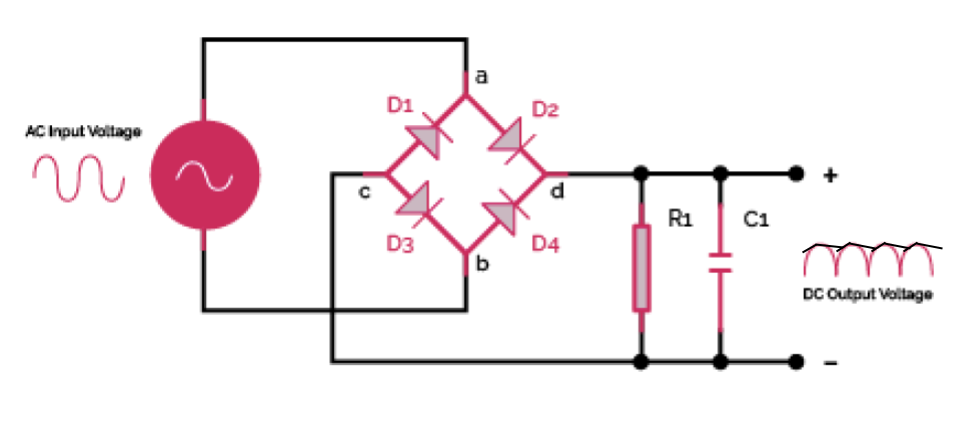# Bridge Rectifier Calculator

Created by Luciano Mino
Last updated: Nov 05, 2022

Our bridge rectifier calculator is the perfect tool to obtain every parameter used in a bridge rectifier, such as current, DC voltage output, RMS current, and ripple factor.

We've paired this full wave bridge rectifier calculator with a brief description of key concepts regarding this topic, such as:

• What a bridge rectifier is;
• How to calculate the output voltage of a bridge rectifier;
• The ripple factor formula and definition;
• More!

## Full wave bridge rectifier definition

A full wave bridge rectifier is a type of electrical circuit that converts alternating current (AC) into direct current (DC). It consists of four diodes arranged in a closed-loop configuration, with each side of this arrangement connected to a different side of the AC supply. As the current passes through the circuit, it experiences minimal ripple effects and produces a relatively stable output voltage.

🙋 If you don't know anything about AC circuits, our RC circuit calculator is the perfect place to start!

## How does a bridge rectifier produce DC from AC?

During the positive half cycle, the diodes D1 and D3 are forward-biased, while D2 and D4 are reverse-biased.

The opposite happens during the negative half cycle: diodes D1 and D3 are reverse-biased, while D2 and D4 are forward-biased.

The result is a unidirectional load current.

We can then reduce the pulsating nature of this current by placing a capacitor in parallel with the resistor.

💡 See our capacitor energy calculator and resistor wattage calculator to understand how capacitors and resistors work.

## How to calculate the output voltage of a bridge rectifier

To calculate the output voltage of a bridge rectifier, you can either use this bridge rectifier calculator or use the following formula:

$\quad V_{DC} = \frac{2 \cdot V_{M}} {\pi}$

where:

• $V_{DC}$ is the average output voltage; and
• $V_{M}$ is the AC peak voltage.

## Ripple factor formula and other parameters

Likewise, you can obtain the load current, ripple factor, and RMS current using this bridge rectifier calculator or by hand using their formulas.

### Ripple factor:

${\footnotesize \text{Ripple factor}} = \sqrt{\Big(\frac{I_{RMS}}{I_{DC}}\Big)^2-1}$

where $I_{RMS}$ is the RMS current and $I_{DC}$ is the average load current.

$\quad I_{DC} = \frac{V_{DC}}{(2R_f + R_L)}$

where:

• $R_L$ is the load resistance;
• $R_F$ is the forward resistance of the diodes; and
• $V_{DC}$ is the output voltage.

### RMS current:

$\quad I_{RMS} = \frac{I_{M}}{\sqrt2}$

where $I_{M}$ is the peak load current.

Luciano Mino
Calculate
Select...Full-wave bridge rectifier diagram.
People also viewed…

### Capacitive reactance

Use our capacitive reactance calculator to find the capacitive reactance of a capacitor in an AC circuit.

### Ideal transformer

The ideal transformer calculator determines the primary or secondary voltage and current based on the number of windings.